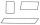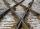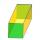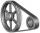# Right triangle - examples - page 7

1. Cut and coneCalculate the volume of the rotation cone which lateral surface is circle arc with radius 15 cm and central angle 63 degrees.
2. Hypotenuse and heightIn a right triangle is length of the hypotenuse c = 56 cm and height hc = 4 cm. Determine the length of both trangle legs.
3. SquareCalculate area of the square with diagonal 64 cm.
4. RT - hypotenuse and altitudeRight triangle BTG has hypotenuse g=117 m and altitude to g is 54 m. How long are hypotenuse segments?
5. RhomboidCalculate the circumference and area of the rhomboid with sides 20 and 10, with their angle 20°.
6. SlopeWhat is the slope of a line with an inclination -221°?
7. SteepleSteeple seen from the road at an angle of 75°. When we zoom out to 25 meters, it is seen at an angle of 20°. What is high?
8. PyramidPyramid has a base a = 5cm and height in v = 8 cm. a) calculate angle between plane ABV and base plane b) calculate angle between opposite side edges.
9. n-gonWhat is the side length of the regular 5-gon inscribed in a circle of radius 12 cm?
10. HeightIs right that in any right triangle height is less or equal half of the hypotenuse?
11. Hexagonal pyramidCalculate the volume and the surface of a regular hexagonal pyramid with a base edge length 3 cm and a height 5 cm.
12. Right triangleCalculate the missing side b and interior angles, perimeter and area of ​​a right triangle if a=10 cm and hypotenuse c = 16 cm.
13. Two forcesTwo forces with magnitudes of 25 and 30 pounds act on an object at angles of 10° and 100° respectively. Find the direction and magnitude of the resultant force. Round to two decimal places in all intermediate steps and in your final answer.
14. RhombusInternal angles of rhombus is in ratio 2:3. How many times is the shorter diagonal longer than side of rhombus?
15. Track arcTwo straight tracks is in an angle 74°. They will join with circular arc with radius r=1127 m. How long will be arc connecting these lines (L)? How far is the center point of arc from track crossings (x)?
16. Airplane navigationAn airplane leaves an airport and flies due west 120 miles and then 150 miles in the direction S 44.1°W. How far is the plane from the airport (round to the nearest mile)?
17. Perimeter and legsDetermine the perimeter of a right triangle if the length of one leg is 75% length of the second leg and its content area is 24 cm2.
18. PrismThe lenght, width and height of a right prism are 6, 17 and 10 respectively. What is the lenght of the longest segment whose endpoints are vertices of the prism?
19. House roofThe roof of the house has the shape of a regular quadrangular pyramid with a base edge 17 m. How many m2 is needed to cover roof if roof pitch is 57° and we calculate 11% of waste, connections and overlapping of area roof?
20. V-beltCalculate the length of the belt on pulleys with diameters of 105 mm and 393 mm at shaft distance 697 mm.

Do you have an interesting mathematical example that you can't solve it? Enter it, and we can try to solve it.

To this e-mail address, we will reply solution; solved examples are also published here. Please enter e-mail correctly and check whether you don't have a full mailbox.

See also our right triangle calculator.